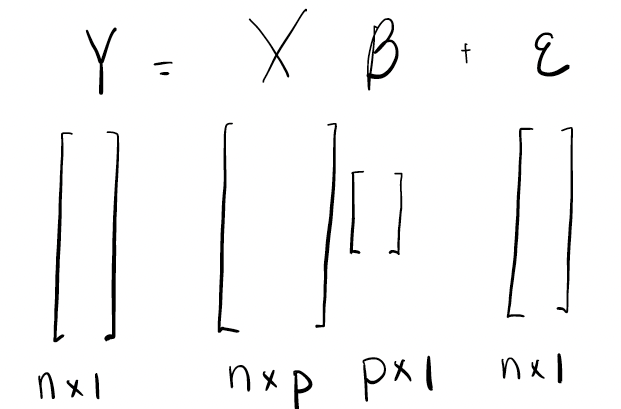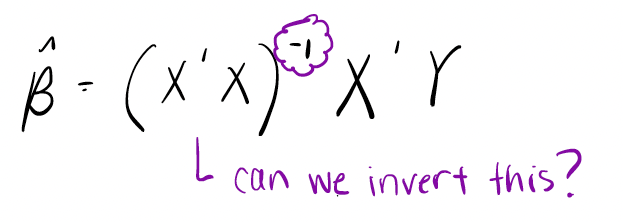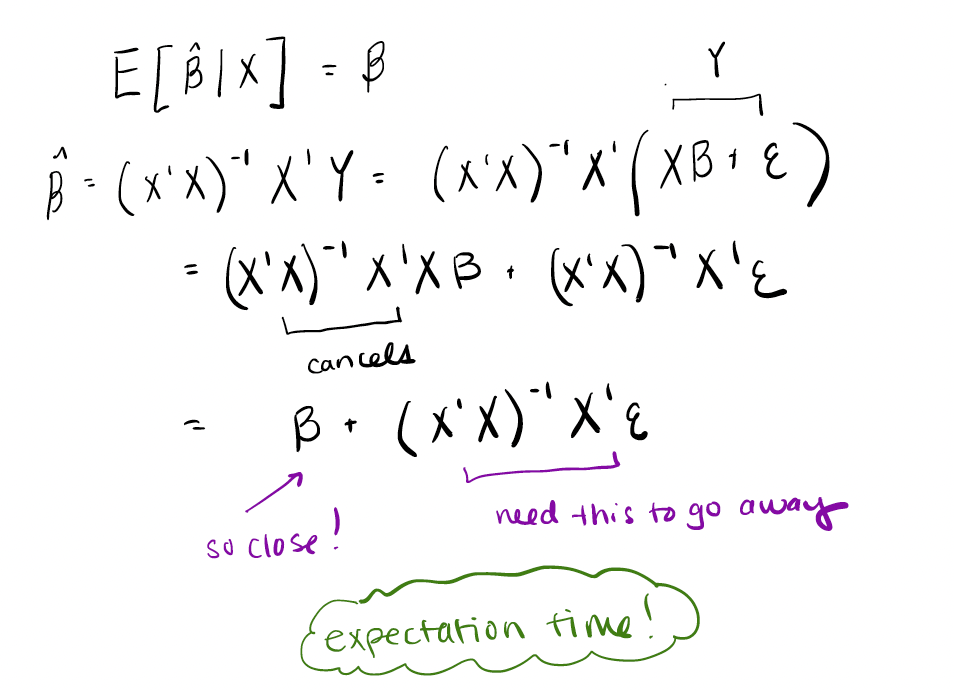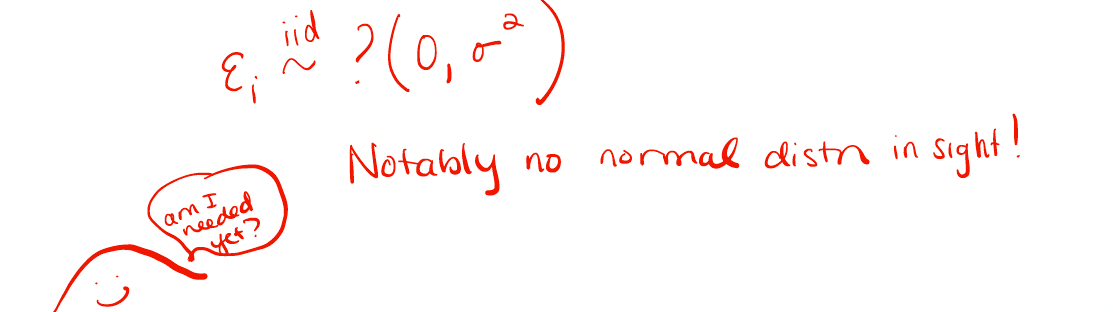# The Origins of Ordinary Least Squares Assumptions

When we start to think more about it, more questions arise. What makes a line “good”? How do we tell if a line is the “best”?

# The Origins of Ordinary Least Squares Assumptions

## Some Are More Breakable Than Others

Sara Stoudt
Bucknell University

#### Introduction

Fitting a line to a set of points… how hard can it be? When those points represent the temperature outside and a town’s ice cream consumption, I’m really invested in that line helping me to understand the relationship between those two quantities. (What if my favorite flavor runs out?!) I might even want to predict new values of ice cream consumption based on new temperature values. A line can give us a way to do that too. But when we start to think more about it, more questions arise. What makes a line “good”? How do we tell if a line is the “best”?

A technique called ordinary least squares (OLS), aka linear regression, is a principled way to pick the “best” line where “best” is defined as the one that minimizes the sum of the squared distances between the line and each point. We chant the assumptions of OLS and know what to look for in diagnostic plots, but where do these assumptions come from? Are there assumptions that are more hurtful than others if broken? These questions are something I even second guess myself, though I frequently use and teach linear regression.

### Bring on the Math

While proving “good” performance of OLS, we need to make certain assumptions to streamline the process. Walking through the proof reveals what assumptions are most crucial and what the impact is of breaking each one.

Let’s first write out our theoretical model in matrix notation and recall what the dimensions of each piece of the puzzle are. We are looking for a linear relationship between $X$ and $Y$, but we know there may be some error, which we represent by $\epsilon$.

#The OLS approach estimates the unknown parameters $\beta$ by $\hat{\beta} = (X’X)^{-1}X’Y$. Here $X’$ is the transpose of $X$, so the product $X’X$ is a square matrix.

#Have we implicitly assumed anything yet? Actually yes, there is an inverse in play! What would $X’X$ have to look like to be invertible? Let’s put our linear algebra hats on.

This matrix would have to be “full rank”. Informally, that means that there is no redundant information in the columns of $X$. So if the number of predictor variables $p$ is greater than the number of data points $n$, that would be a problem. Even if $p < n$, the columns of $X$ still need to be linearly independent. Multicollinearity (when one covariate is highly correlated with another covariate) would make us suspicious of this. Let’s keep that in mind just in case it comes back to haunt us.

Now that we have our estimator for $\beta$, there are two properties we want to check on.

1. We want to on average get the “right” answer.
2. We want to be able to describe the uncertainty in our estimate. In other words, how much would our estimate wiggle around if we happened to have a new sample?

Let’s start with determining if we “expect” to get the right answer on average. It will help to rewrite $Y$ based on the proposed model.

#As we break this expression down, we can see that we are on the right track. However, somehow that second term needs to have expectation zero.

#How can we make this happen? This is where two regression assumptions are born. First we need the errors, $\epsilon$, to be independent of $X$. This seems plausible. If the errors depend on $X$, somehow we still have some information leftover that is not accounted for in the model. If the errors did depend on $X$, that would be a form of heteroscedasticity (non-constant variance for short). That sets our OLS assumption alarm bells ringing. We also need to make an assumption about the magnitude of the errors themselves. It would be nice if they were not systematically positive or negative (that doesn’t seem like a very good model), so assuming they are on average zero seems like a reasonable path forward.

#With these assumptions in hand we now have an estimator that is unbiased. We expect to get the right answer on average. So far we have seen the motivation for two assumptions. If either of those are broken, this unbiasedness is not guaranteed. So if our goal is to make predictions or interpret these coefficients in context, we will be out of luck if these assumptions aren’t met. The next step is to understand the estimator’s uncertainty. Let’s see what other assumptions reveal themselves in the process.

#So let’s recap what we’ve had to assume about the errors so far.

#What haven’t we had to assume yet? Normality! Then why is everyone always worried about that when doing regression? Why all of those qqplots?! Well, we do need to know the whole sampling distribution of the estimates if we want to do inference, or say something about a more general population based on a sample. If we assume the distribution that those errors have is actually Normal, that lets us get a normal sampling distribution of $\hat{\beta}$.

#Ah, but there is that pesky inverse again! If we have multicollinearity, that inverse might get unstable, affecting our understanding of the spread of the sampling distribution. And of course nothing in life is perfectly normal. However, we can often get an approximately normal sampling distribution if our sample size is large enough thanks to the Central Limit Theorem (an explainer for another time), so there is some robustness to breaking this particular assumption.

We’ve now seen all of the regression assumptions unfold, so we should be able to build a sort of hierarchy of assumptions.

#This hierarchy is also borne out in simulation studies like this one that try to stress test OLS. You may even want to code up a simple simulation yourself if you want to look for any gaps between the theory and practice.

Some broken assumptions are fixable though. We might transform variables to deal with a lack of linearity or use a Generalized Linear Model that allows the relationship between $X$ and $Y$ to take a non-linear form. We might just be happy with estimating the best linear projection of the true relationship. If errors aren’t independent and/or do not have constant variance, methods like generalized least squares (GLS) can step in. If errors are not normally distributed, they might be approximately normally distributed thanks to the Central Limit Theorem, or we might want to use a Generalized Linear Model (GLM) that can handle error distributions of other forms. We always have options!

It’s great to see the theory behind the scenes informing the practice, and in general, assumptions of any method have to come from somewhere. Seeking out where in the math assumptions help make our lives easier can help demystify where these assumptions actually come from and gives some insight into which ones, if broken, are more dangerous than others. Happy line fitting!

### All the Math in One Place

$Y = X \beta + \epsilon$

$\hat{\beta} = (X’X)^{-1}X’Y$

$E[\hat{\beta} | X] = \beta$

$\hat{\beta} = (X’X)^{-1} X’ Y = (X’X)^{-1} X’ (X\beta + \epsilon)$

$= (X’X)^{-1} X’X\beta + (X’X)^{-1} X’\epsilon$

$= \beta + (X’X)^{-1}X’ \epsilon$

$E[\hat{\beta} | X] = E[(\beta + (X’X)^{-1} X’ \epsilon) | X]$

$= E[\beta | X] + E[(X’X)^{-1}X’ \epsilon | X]$

$= \beta + (X’X)^{-1} X’ E[\epsilon | X]$

$E[\epsilon | X] = 0?$

$\epsilon_i \stackrel{iid}{\sim} N(0, \sigma^2)$

$\hat{\beta} \sim N(\beta, \sigma^2 (X’X)^{-1})$

### References

• Statistical Models: Theory and Practice by David A. Freedman, Cambridge University Press (2009)
• Mostly Harmless Econometrics: An Empiricist’s Companion by Joshua D. Angrist and Jörn-Steffen Pischke, Princeton University Press (2009)

Special thanks to my students in my Stat 2 class this semester for raising good questions about these regression assumptions and to my colleagues for helping me work through this hierarchy before reporting back. Also, thanks to Features Writer Courtney R. Gibbons for including drawings in her last post. That inspired me to do some doodling myself.

Note: The website https://drawdata.xyz/ made it possible to generate data to make the plots in the opening image.